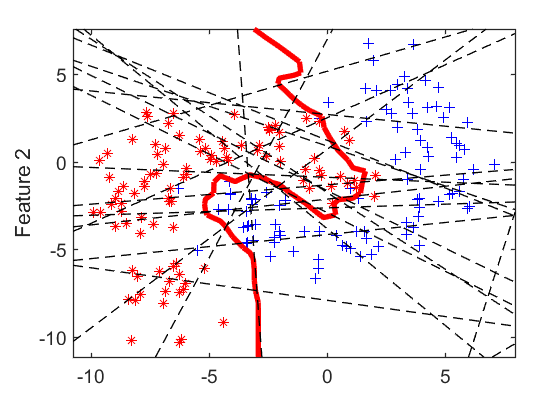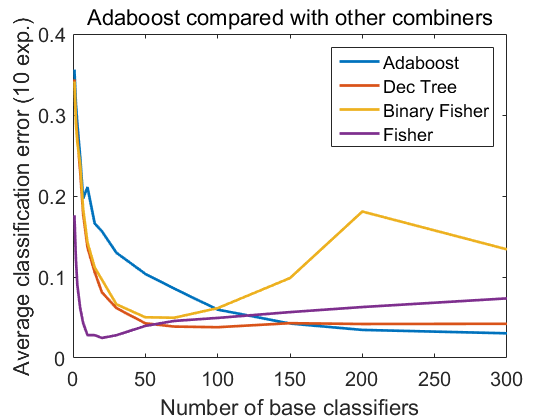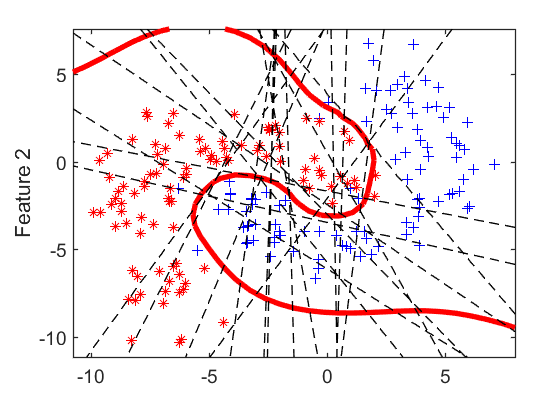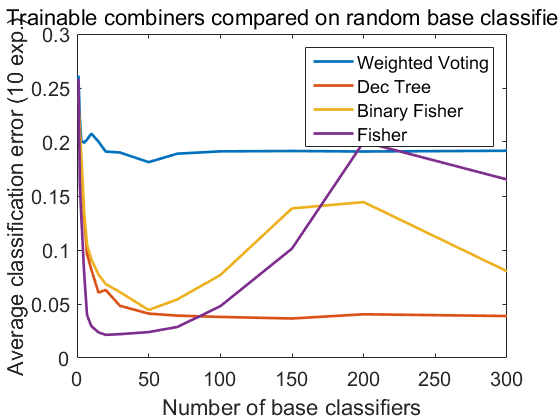Adaboost is compared with other base classifier generators and combiners.

PRTools should be in the path

Download the m-file from here. see http://37steps.com/prtools for more.

## Initialisation

```randreset(1000);
t = gendatb([10000 10000]);
N = [1 2 3 5 7 10 15 20 30 50 70 100 150 200 300];
nrep = 10;
e1 = zeros(4,numel(N),nrep);
e2 = zeros(4,numel(N),nrep);
delfigs
prtime(inf); % switch off prtime
```

## Adaboost base classifiers: computation and combination

N base classifiers based on single epoch linear percoptron are trained for N = 1 ... 300. They are combined in various ways: * standard Adaboost weights * decision tree * Fisher based on the binary outcomes of the base classifiers * Fisher based on the confidence outcomes of the base classifiers Classification errors are computed for 10 repititions.

```for i=1:nrep
randreset(i);
a = gendatb([100 100]);
nclassf = numel(w.data{2});
v = w.data{1}.data;
u = w.data{2};
for j=1:numel(N)
n = N(j);
w1 = wvotec(stacked(v(1:n)),u(1:n));
w2 = a*(stacked(v(1:n))*mapm('ge',0.5)*dtc);
w3 = a*(stacked(v(1:n))*mapm('ge',0.5)*fisherc);
w4 = a*(stacked(v(1:n))*fisherc);
e1(:,j,i) = cell2mat(testc(t,{w1 w2 w3 w4}))';
end
end
```

## Adaboost classifier example

The first 20 base classifiers are shown and the resulting Adaboost classifier based on 300 base classifiers.

```figure;
scatterd(a);
plotc(w,'r',4);
plotc(v(1:20),'k--',1); legend off
title('The problem, the first 20 base classifiers, the final Adaboost')
fontsize(14)
```## Learning curves for increasing numbers of Adaboost generated base classifiers

```figure;
plot(N,mean(e1,3)')
title('Adaboost compared with other combiners')
xlabel('Number of base classifiers')
ylabel(['Average classification error (' num2str(nrep) ' exp.)'])
fontsize(15);
linewidth(2);
```## Random base claasifiers

Instead of the Adaboost incrementally computed base classifiers, now a set of N (1 ... 300) base classifiers is generated by the 1-NN rule based on a randomly chosen single objects per class. They are combined in various ways: * weighted voting, similar to Adaboost, but based on the performance of the entire training set. * decision tree * Fisher based on the binary outcomes of the base classifiers * Fisher based on the confidence outcomes of the base classifier

```for i=1:nrep
randreset(i);
a = gendatb([100 100]);
v = a*repmat({gendat([],[1 1])*knnc([],1)},1,300);
w = wvotec(a,stacked(v));
u =w.data{2};
for j=1:numel(N)
n = N(j);
w1 = wvotec(stacked(v(1:n)),u(1:n));
w2 = a*(stacked(v(1:n))*mapm('ge',0.5)*dtc);
w3 = a*(stacked(v(1:n))*mapm('ge',0.5)*fisherc);
w4 = a*(stacked(v(1:n))*fisherc);
e2(:,j,i) = cell2mat(testc(t,{w1 w2 w3 w4}))';
end
end
```

## Random Fisher combiner example

The first 20 random base classifiers are shown and the resulting Fisher combiner based on just these 20 base classifiers.

```figure;
scatterd(a);
plotc(a*(stacked(v(1:20))*fisherc),'r',4)
plotc(v(1:20),'k--',1); legend off
title('The problem, the first 20 base classifiers combined by Fisher')
fontsize(14)
```## Learning curves for increasing numbers of randomly generated base classifiers

```figure;
plot(N,mean(e2,3)')
legend('Weighted Voting','Dec Tree','Binary Fisher','Fisher')
title('Trainable combiners compared on random base classifiers')
xlabel('Number of base classifiers')
ylabel(['Average classification error (' num2str(nrep) ' exp.)'])
fontsize(15);
linewidth(2);
```